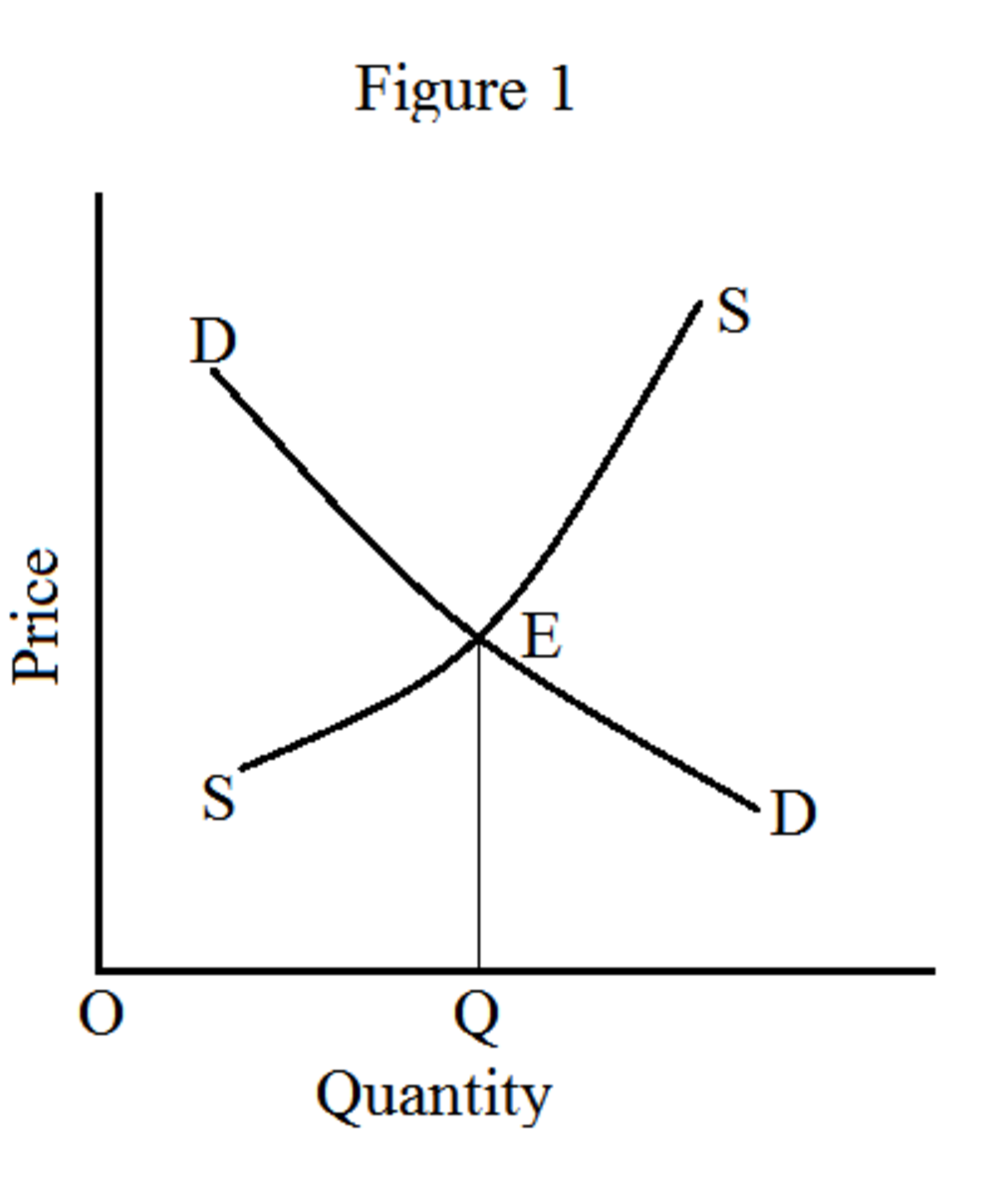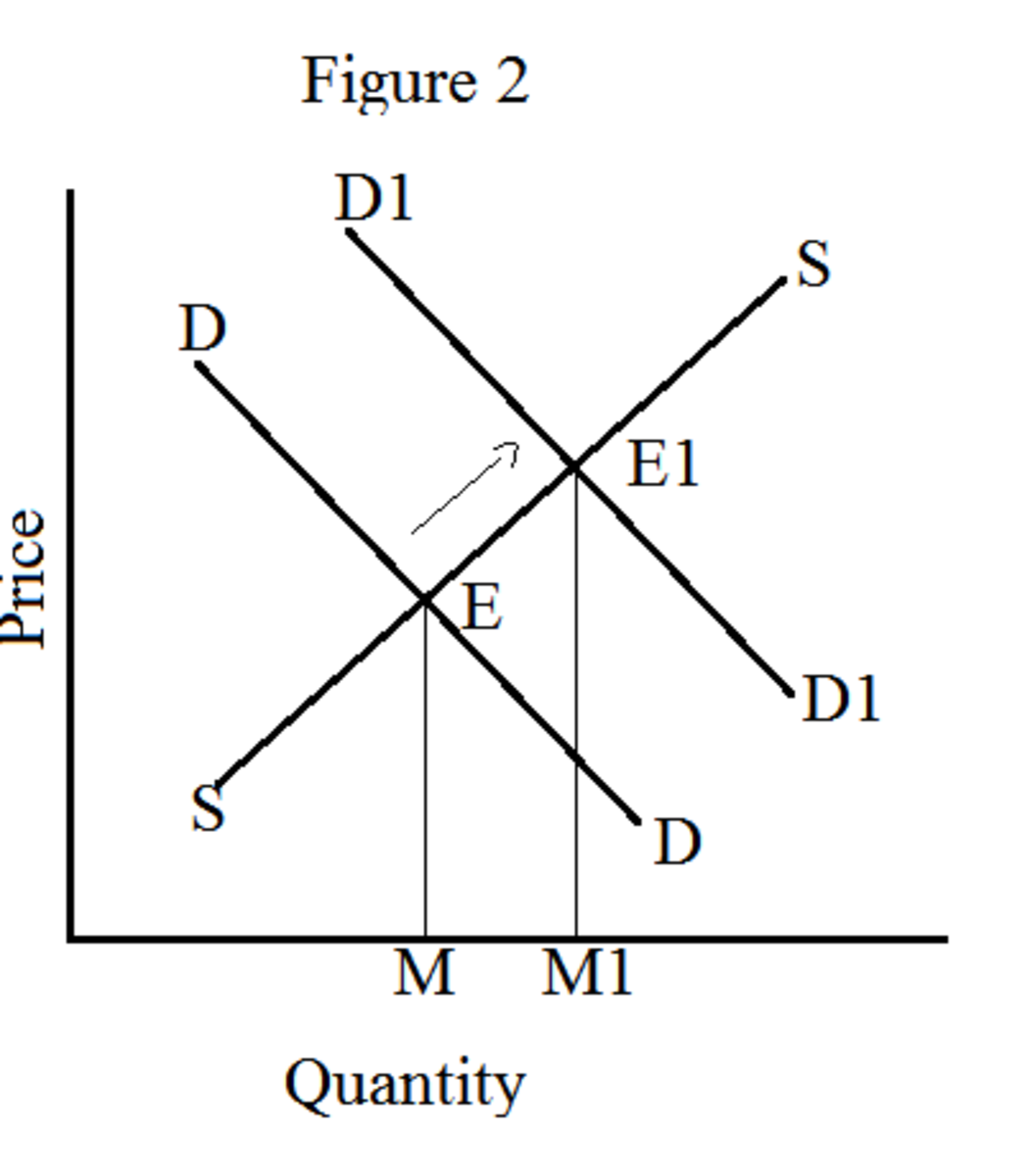Updated date:

# Static and Dynamic Equilibrium

## Introduction

The terms ‘statics’ and ‘dynamics’ are widely used in physical sciences. In fact, a theoretical physicist called August Comte introduced these concepts to social sciences. The term ‘statics’ is derived from a Greek word called ‘Statikos’. Statikos means ‘causing to stand’. The term ‘dynamics’ is also derived from a Greek word called ‘Dynamikos’. Dynamikos means ‘causing to change.’ Though J.S. Mill attributed the application of these concepts in economics, the distinction between economic statics and economic dynamics was vague. However, Prof. Ragner Frisch resolved this issue and made a scientific distinction between the two terms.

## Economic Statics

Though the term ‘statics’ is used in both physical sciences and economics, its meaning differs substantially. In physical sciences, statics refers to a state in which there is no change in the position. In other words, statics refers to a state of rest. For instance, we can say an object lying on the floor without any motion is in static state. In economics, statics gives a very different meaning. Economic statics does not mean that there is no economic activity. Economic activities occur. Economic statics is basically an analysis of economic variables during the specified time. All economic variables under consideration relate to the same point of time. In other words, what we try to analyze in economic statics is that we establish functional relationship among economic variables whose values belong to the same time.

Furthermore, economic statics is concerned with the equilibrium values only. It does not study about the path of equilibrium. For instance, suppose ‘A’ is an equilibrium and ‘B’ is the new equilibrium. Economic statics is concerned with equilibrium points ‘A’ and ‘B’, and does not study the path from ‘A’ to ‘B’. Hence, time element has no role in economic statics.Let us look at figure 1. The diagram portrays equilibrium price determination under perfect competition. The equilibrium is established at the point where demand curve DD and supply curve SS intersect. QE and OQ are the equilibrium price and equilibrium quantity respectively. In this analysis, the variables involved to determine equilibrium are price, quantity demanded and quantity supplied. Note that all these variables relate to the same time (the time when the equilibrium is established). Because of this reason, this is known as static analysis. At this particular time, aforementioned variables act as constants. Monopolistic competition of Chamberlin and imperfect competition of Joan Robinson’s are good examples of economic statics.

## Comparative Statics

Comparative statics is nothing but comparing two equilibrium positions. Suppose ‘A’ is original equilibrium point. ‘B’ is the new equilibrium point. Under comparative statics, we compare equilibrium ‘A’ and ‘B’. And we do not study the path of equilibrium, i.e. how new equilibrium ‘B’ is established from ‘A’.For example, in figure 2, DD represents original demand curve and SS represents original supply curves. The intersection of DD and SS establishes point of equilibrium E. At this equilibrium, OM is the quantity and ME is the price. Now a new demand curve D1D1 forms due to change in the determinants of demand. This establishes new equilibrium E1. The new equilibrium price is M1E1 and new equilibrium quantity is OM1. In comparative statics, we just compare the original equilibrium price ME and the new equilibrium price M1E1. Likewise, we compare the original equilibrium quantity OM and the new equilibrium quantity OM1. Note that the path of change (from E to E1) is not under the purview of this analysis.

## Economic Dynamics

After reading economic statics and comparative statics, you are able to guess easily what economic dynamics is all about. Economic dynamics studies the path of equilibrium or evolutionary process. Suppose that the original equilibrium is ‘A’ and the new equilibrium is ‘B’. Under economic dynamics, we study how the new equilibrium ‘B’ is attained. Between equilibrium points ‘A’ and ‘B’, all economic variables under consideration keep changing over time. Therefore, the variables are considered as functions of time. Hence, time element plays a vital role in economic dynamics.

Economic dynamics is more complicated because it includes all changes, sequences, lags and even expectations in the analysis. At the same time, this is more realistic since major economic factors such as size of population, supply of capital, methods of production, forms of business organization and human wants are dynamic in nature. Furthermore, the study of economic dynamics avoids unrealistic assumptions such as constant tastes and preferences.

## Economic Statics Vs. Economic Dynamics

Economic StaticsEconomic Dynamics

There is no role for time element.

Time element plays a vital role.

This does not study the path of equilibrium or evolutionary process.

Dynamic analysis studies the evolutionary process.

Static analysis is based on assumptions such as ceteris paribus. Some of the assumptions are very unrealistic.

Economic dynamics avoids unrealistic assumptions.

Economic statics is based on deductive analysis.

Economic dynamics is based on inductive analysis.

Inspite of unrealistic assumptions, major economic theories are based on economic statics.

Though realistic and comprehensive, its application is limited. For instance, it fails to support growth theories of economics.

© 2013 Sundaram Ponnusamy

## Comments

shilpa das. on September 06, 2017:

It is so helpful for me...